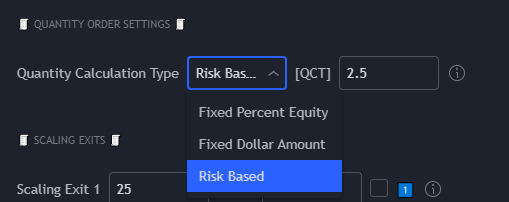Prism Bot and Prism Strategies has several methods for calculating your quantity.

You can use any of the methods outlined here to calculate how much you are risking while trading.

When selecting these options, Prism Bot will receive the exact values for both Fixed Dollar Amount and Fixed Percent Equity (which will calculate from your exchange capital available).However, Risk Based works a bit differently. Instead, this option will calculate based on the stop loss.

The formula for this is as follows:

Order Size = Equity * Risk % / Stop Loss %

This formula demonstrates that your risk of your equity is always the same, and only changes the order size based on the stop loss value.

This method is a compounding method for quantity calculations, and is the default method by which the bot will calculate your order sizes.

NOTE: PrismBot does not rely on Tradingview contract quantities to calculate your position sizes. The bot will read the equity available on your exchange and use that value instead. The Tradingview contract sizes are demonstration and backtester results only. If you do wish to use the contracts calculated by Tradingview, it is recommended to use "Send Contract Quantities by Strategy Calculation". However, this feature is not necessary if you prefer the bot to use the equity available on your exchange account.